Open in App
Not now

# How to Find Interior and Exterior Skeleton of Binary Images Using MATLAB?

• Difficulty Level : Medium
• Last Updated : 18 Jan, 2022

Skeletonization is a process for reducing foreground regions in a binary image to a skeletal remnant that largely preserves the extent and connectivity of the original region while throwing away most of the original foreground pixels. In this article, we will see how to find interior and exterior skeleton of binary images using MATLAB in-built function.

Thinning: Thinning is a morphological operation performed on binary images. It removes some selected foreground pixels from binary images and hence thins the image. Mathematically, it is the difference of binary image and the result of hit-or-miss transformation applied on the same binary image.

• Inner Skeleton of image: Every binary image can be thinned infinite times. The inner structure is obtained when thinning operation is applied infinite times until the resultant image is 1 pixel thick. The underlying inner skeleton of the binary image is obtained finally.
• Exterior Skeleton of image: The exterior skeleton of the image consists of the boundary only and interior pixels of the image are removed. It is different from edge detection because in edge detection we detect all interior edges along with boundary edges. In the exterior skeleton finding the result only consist of the outer boundary and all interior pixels are removed.

Function Used:

• rgb2gray( ) inbuilt function is used to convert RGB colour image into grayscale.
• bwmorph( ) inbuilt function is used to find skeleton of the image.
• imtool( ) inbuilt function is used to display the image.

Example:

## Matlab

 `% Find Skeleton of a binary image.``% read the image.``k=imread(``'skeleton.png'``);` `% Convert into grayscale.``k=rgb2gray(k);` `% apply morphological operation.``k1=bwmorph(k,``'skel'``,Inf);` `% display original image.``imtool(k);` `% display skeleton of image.``imtool(k1);`

Output: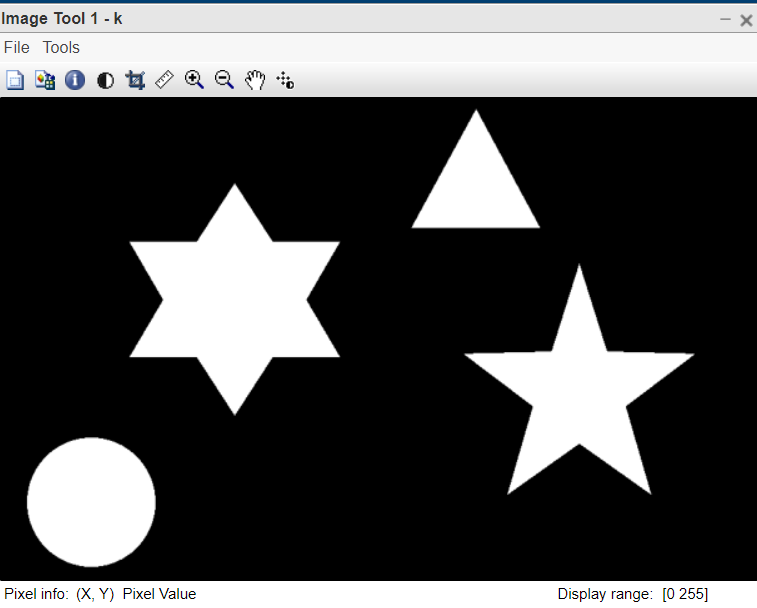Figure 1: Input image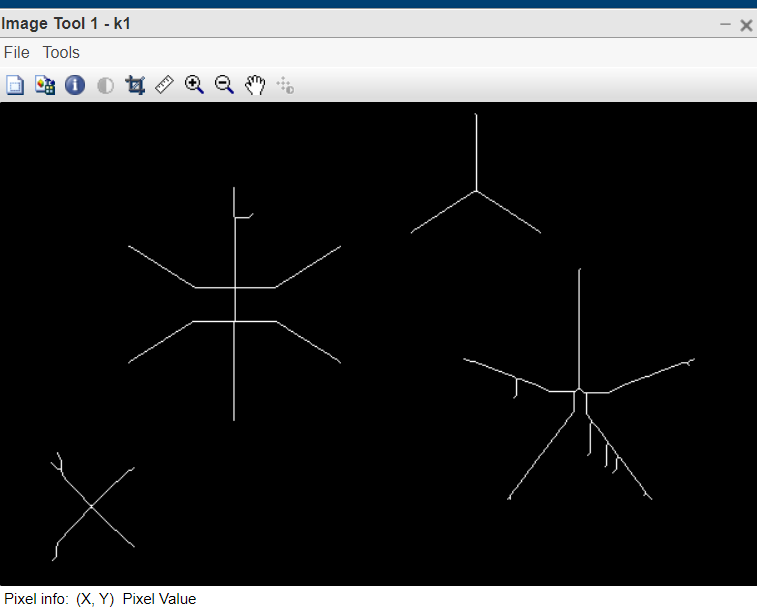Figure 2:Output image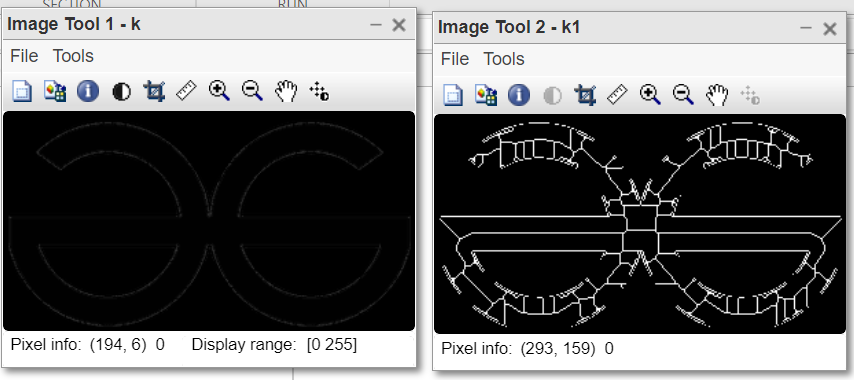Figure 3: Results on GFGlogo

Example:

## Matlab

 `% MATLAB code for remove``% inner pixels of image.``% read the image.``k=imread(``'skeleton.png'``);` `% Convert into grayscale.``k=rgb2gray(k);` `% Apply morphological operation.``k2=bwmorph(k,``'remove'``,Inf);` `% display original image.``imtool(k);` `% display boundary image.``imtool(k2);`

Output:Figure 4: Input image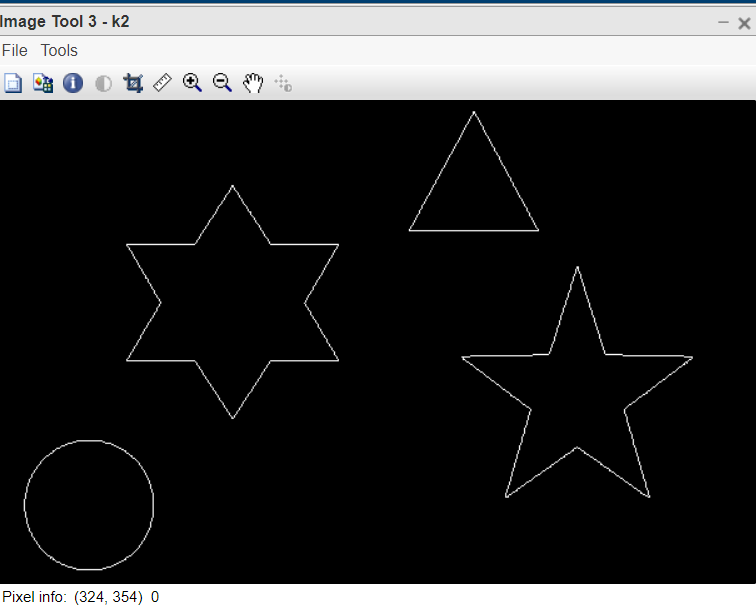Figure 5: Output image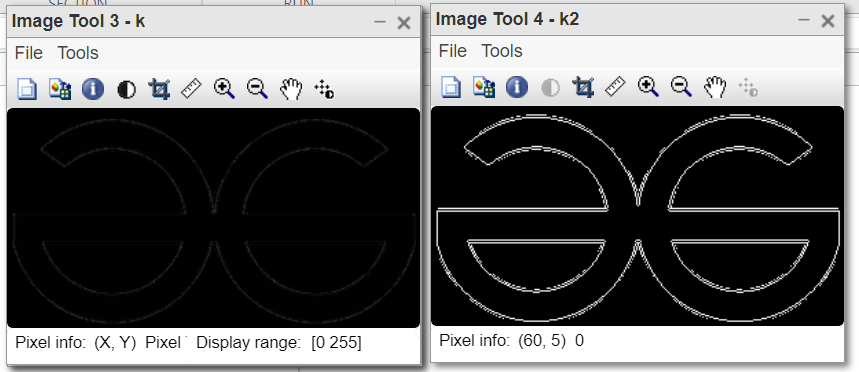Figure 6: Result on GFGlogo

My Personal Notes arrow_drop_up